# Examples for 7th grade (seventh)

#### Number of examples found: 1974

• Rotating coneCalculate volume of a rotating cone with base radius r=12 cm and height h=7 cm.
• Rhombus anglesIf one angle in the rhombus is 159°, what is it neighboring angle in rhombus?
• EqnSolve equation with fractions: 2x/3-50=40+x/4
• Length subtractingExpress in mm: 5 3/10 cm - 2/5 mm
• Strange x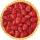For what x is true ??
• Lcd3What is LCD of the equation of ? ? And what is x?
• Isosceles trapezoid v3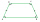In an isosceles trapezoid ABCD is the size of the angle β = 81° Determine size of angles α, γ and δ.
• Feet to milesA student runs 2640 feet. If the student runs an additional 7920 feet, how many total miles does the student run?
• The quotientThe quotient of g and 55 is the same as 279. What is g?
• One hectareHow many square meters are one hectare?
• EquationSolve the equation: 1/2-2/8 = 1/10; Write the result as a decimal number.
• Missing numberBlank +1/6 =3/2 find the missing number
• Square prismA square prism has a base with a length of 23 centimeters, what is the area in square centimeters of the base of the prism?
• A numberA number increased by 7.9 is 8.3
• CubeThe sum of all cube edges is 30cm. Find the surface area of the cube.
• Write 2Write 791 thousandths as fraction in expanded form.
• 9-gon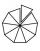Sum of interiol angles of 9-gon is:
• Equation 15Solve equation with variables on both sides:
• One sixth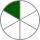How many sixths are two thirds?
• Rhombus HP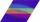Calculate area of the rhombus with height 24 dm and perimeter 12 dm.

Do you have an interesting mathematical word problem that you can't solve it? Submit a math problem, and we can try to solve it.

We will send a solution to your e-mail address. Solved examples are also published here. Please enter the e-mail correctly and check whether you don't have a full mailbox.

Please do not submit problems from current active competitions such as Mathematical Olympiad, correspondence seminars etc...